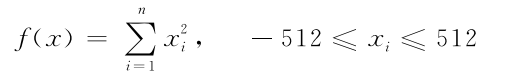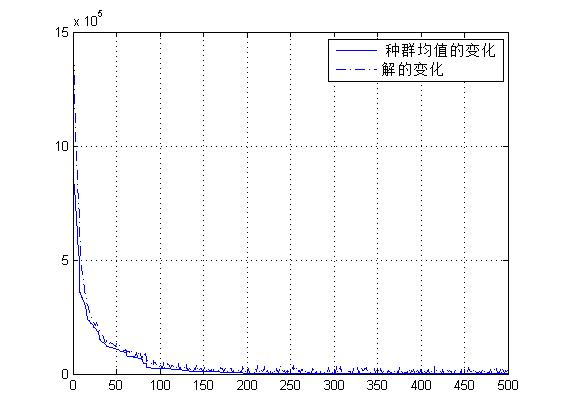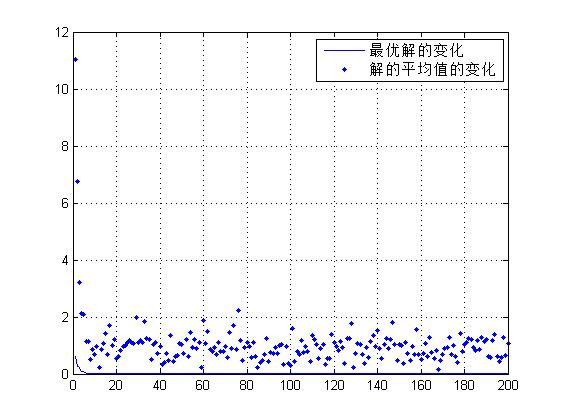• 实验五 用matlab求二元函数的极值 1计算二元函数的极值 对于二元函数的极值问题,根据二元函数极值的必要和充分条件,可分为以下几个步骤: z?f(x,y. 定义二元函数步骤1.f(x,y?0,f(x,y?0yx,得到驻点. 步骤2.求解方程组...
• 下列二元函数的最大值，f(x1,x2)=x1^2+x2^2,x1与x2的取值区间为{0,1,2，...，7}
• 今天小编就为大家分享一篇使用遗传算法求二元函数最小值，具有很好的参考价值，希望对大家有所帮助。一起跟随小编过来看看吧
• 本文件使用粒子群算法计算出二元函数最小值，运行速度快。得到的最小值非常接近最优值。只需修改对应的函数表达式，根据所需要的区间，即可运行
• %% 学习目标：一元函数最小值和零点 %% 函数的极小值和零点 求解一元函数的最小值 clear all; close all; [x,fval]=fminbnd(@cos,1,5) %函数句柄 y=cos(x) %% 求解自变量范围限定的一元函数的最小值 clear all; ...
%% 学习目标：求一元函数最小值和零点 %% 函数的极小值和零点 求解一元函数的最小值 clear all; close all; [x,fval]=fminbnd(@cos,1,5) %函数句柄 y=cos(x) %% 求解自变量范围限定的一元函数的最小值 clear all; close all; f=@(x)x.^2-6x-9; [x,fval]=fminbnd(f,0,6,optimset(‘Display’,‘iter’)) %自变量在0到6之间的最小值，显示计算过程 y=f(x) %% 求多元函数的最小值 clear all; close all; f=@(x)2(x(2)-x(1)2)2+(2-x(1))^2; [x,fval]=fminsearch(f,[-6.7 8]) %指定一个起始点 在某点附近寻找 y=f(x) %% 求解一元函数的零点 clear all; close all; f=@(x)sin(x)*cos(x); figure; fplot(f,[-5,5]); xlabel(‘x’); ylabel(‘f(x)’); grid on; x1=fzero(f,[-4,-2]) x3=fzero(f,-2) [x4,fval]=fzero(f,[-3,-1])
新上线了一门视频课程——二十八天精通matlab基础编程-在线视频教程-CSDN学院 https://edu.csdn.net/course/detail/24947
展开全文• 一节课精通matlab入门一元二元函数最小值和零点，包括详细的代码资料和讲解注释
• 多元函数表达式如下： 其最小值显然在0处%定义遗传算法参数 NIND=40; %个体数目(Numbe of individuals) MAXGEN=500; %最大遗传代数(Maximum number of generations) NVAR=20; %变量的维数 PRECI
本文基于谢菲尔德大学遗传算法工具箱。  多元函数表达式如下：其最小值显然在0处
%定义遗传算法参数
NIND=40;               %个体数目(Numbe of individuals)
MAXGEN=500;            %最大遗传代数(Maximum number of generations)
NVAR=20;               %变量的维数
PRECI=20;              %变量的二进制位数(Precision of variables)
GGAP=0.9;              %代沟(Generation gap)
trace=zeros(MAXGEN, 2);
%建立区域描述器(Build field descriptor)
FieldD=[rep([PRECI],[1,NVAR]);rep([-512;512],[1, NVAR]);rep([1;0;1;1],[1,NVAR])];
Chrom=crtbp(NIND, NVAR*PRECI);                       %创建初始种群
gen=0;                                               %代计数器
ObjV=objfun1(bs2rv(Chrom, FieldD));                  %计算初始种群个体的目标函数值
while gen<MAXGEN                                     %迭代
FitnV=ranking(ObjV);                             %分配适应度值(Assign fitness values)
SelCh=select('sus', Chrom, FitnV, GGAP);         %选择
SelCh=recombin('xovsp', SelCh, 0.7);             %重组
SelCh=mut(SelCh);                                %变异
ObjVSel=objfun1(bs2rv(SelCh, FieldD));           %计算子代目标函数值
[Chrom ObjV]=reins(Chrom, SelCh, 1, 1, ObjV, ObjVSel);     %重插入
gen=gen+1;                                                 %代计数器增加
trace(gen, 1)=min(ObjV);                                   %遗传算法性能跟踪
trace(gen, 2)=sum(ObjV)/length(ObjV);
end
plot(trace(:,1));hold on;
plot(trace(:,2),'-.');grid;
legend(' 种群均值的变化','解的变化')
%输出最优解及其对应的20个自变量的十进制值,Y为最优解,I为种群的序号
[Y, I]=min(ObjV)
X=bs2rv(Chrom, FieldD);
X(I,:)
代码来自于雷英杰《MATLAB_遗传算法工具箱与应用》
注意
与一元函数不同，这里的种群是40*400大小的矩阵，40是种群中个体数目，400是20*20，因为有该多元函数有20个变量，每个变量用20位来表示。、  同时，区域描述器FiledD也要跟着变成20列，每一列描述一个变量。代码运行之后，查看X可以发现，是一个40*20的矩阵，绝大部分都接近于0值，而20个0正好是最小值处。
展开全文遗传算法
• 该实验使用模拟退火算法函数最小值matlab自编程实现。该实验可以观察到搜索点的过程，也可以自行修改参数。
• 利用MATLAB计算任意二元函数的极小值的详细模板 请用pdf打开
• 本代码主要利用MATLAB工具实现MATLAB——求解无约束非线性函数最小值，简单明了，易于理解
• 其原理简单，就求函数最小值这个应用而言，大致说来就是先出该函数梯度，大家都知道梯度是一个函数在该点的最快上升率，那么个相反数就是最快下降率了。将每个自变量按下列公式更新：  xt+1=xt+Δx xt+1=x...
算法原理

梯度下降法是一个最优化算法，可以用来求一个函数的最小值，最大值等，也常用于人工神经网络中更新各个感知器之间的权值，求出cost function的最小值等，应用广泛。  其原理简单，就求函数的最小值这个应用而言，大致说来就是先求出该函数梯度，大家都知道梯度是一个函数在该点的最快上升率，那么求个相反数就是最快下降率了。将每个自变量按下列公式更新：  xt+1=xt+Δx xt+1=xt+Δx
其中  Δx=−η∂f∂x Δx=−η∂f∂x

ηη称为学习速率，也称为步长，指明了自变量朝向最快下降方向减少的速度，这个值的选定相当重要，太大了会导致震荡，无法收敛到最小值点，太小了会导致收敛速度过慢。  当xt+1xt+1和xtxt的差小于一个阀值时，就可以停止循环了。

Matlab实现
% 没有加入冲量项的随机梯度下降法实现
syms x y real
y(x) = x^2+2*x+10 ;
delta(x) = -diff(y(x)) ;
step = 0.1 ;
first_x = 10 ;
x_current = first_x ;
x_next = first_x ;
show_tmp = 0 ;
show = [] ;
counter = 0 ;
delta_last = 0 ;
while (true)
delta_x = double(delta(x_current))*step ;
if(abs(delta_x) < 0.0001)
break
end
x_next = x_current + delta_x ;
x_current = x_next ;
counter = counter + 1;
show_tmp = x_next ;
show = [show, show_tmp] ;
if(counter > 200)
break ;
end
end
x_next
counter
figure(1)
ezplot(y(x))
hold on
plot([-100, 100],[double(y(x_next)), double(y(x_next))],'-r')
double(y(x_next))
figure(2)
plot(show)
pause
close all

为了来定义我们预测值和真实值之间的差异，我们引入代价函数(cost function)的概念所以，现在我们的任务就是来求得一个Θ，从而使得代价函数达到最小，也即全局最小(global minimun).在这里我们使用梯度下降算法来求函数的最小值。

梯度下降法是按下面的流程进行的：
1）首先对θ赋值，这个值可以是随机的，也可以让θ是一个全零的向量。
2）改变θ的值，使得J(θ)按梯度下降的方向进行减少。

如下图：上图表示的是参数Θ和代价函数J(Θ)的关系图，深蓝色为全局最小，浅蓝色为局部最小，红色则表示J(Θ)有一个较大的取值，而梯度下降算法就是我们给定一个初始的Θ值，然后按照梯度下降的原则不断更新Θ值，使得J(Θ)向更低的方向进行移动。算法的结束将是在θ下降到无法继续下降为止。上面两条线代表我们给定两个初值，我们发现一条到达局部最小，即浅蓝色，而一条到达全局最小，即深蓝色。所以从这里我们可以看出，初始值的选择对梯度下降的影响很大。
梯度下降的更新规则：解释：描述：对 θ 赋值，使得 J(θ)按梯度下降最快方向进行，一直迭代下去，最终得到局部最小值。 其中 α 是学习率（ learning rate） ， 它决定了我们沿着能让代价函数下降程度最大的方向向下迈出的步子有多大。
对于梯度下降，我们再来直观的画出图像理解一下，J(Θ)的图像我们如下求导的目的，基本上可以说取这个红点的切线，就是这样一条红色的直线，刚好与函数相切于这一点，让我们看看这条红色直线的斜率，就是这条刚好与函数曲线相切的这条直线，这条直线的斜率正好是这个三角形的高度除以这个水平长度，现在，这条线有一个正斜率，也就是说它有正导数， 因此， 我得到的新的 θ1， θ1 更新后等于 θ1 减去一个正数乘以 α。当Θ取到最小，J(Θ)最小，也即Θ不能小时算法结束。

对于α ，后面会讲到，这里先简单提下：
如果 α 太小了，即我的学习速率太小，结果就是只能这样像小宝宝一样一点点地挪动，去努力接近最低点，这样就需要很多步才能到达最低点，所以如果 α 太小的话，可能会很慢因为它会一点点挪动，它会需要很多步才能到达全局最低点。 如果 α 太大，那么梯度下降法可能会越过最低点，甚至可能无法收敛， 下一次迭代又移动了一大步，越过一次，又越过一次，一次次越过最低点，直到你发现实际上离最低点越来越远，所以，如果 α 太大，它会导致无法收敛，甚至发散。

当只有一个训练样本时，执行梯度下降算法（注意这里只是对Θj求导，所以最后乘上的是xj）所以针对一个训练样本，更新规则如下：现在推广到存在多个特征时注意：迭代都是需要同时进行的。在进行随机梯度下降算法时，我们每次迭代都只选取一个训练样本，这样当我们迭代到若干样本的时候Θ就已经迭代到最优解了。

展开全文• 二元函数为y=x1^2+x2^2,x∈[-5,5]
二元函数为y=x1^2+x2^2,x∈[-5,5]
NIND=121;       %初始种群的个数(Number of individuals)
NVAR=2;         %一个染色体（个体）有多少基因
PRECI=20;       %变量的二进制位数(Precision of variables)
MAXGEN=200;     %最大遗传代数(Maximum number of generations)
GGAP=0.8;       %代沟(Generation gap)，以一定概率选择父代遗传到下一代
trace=zeros(MAXGEN,2);          %寻优结果的初始值

Chrom=crtbp(NIND,PRECI*NVAR);   %初始种群

%区域描述器(Build field descriptor)
%确定每个变量的二进制位数，取值范围，及取值范围是否包括边界等。
FieldD=[rep([PRECI],[1,NVAR]);rep([-5;5]，[1,NVAR]);rep([1;0;1;1],[1,NVAR])];
Objv=objfun(bs2rv(Chrom,FieldD))
gen=1;                  %代计数器
while gen<=MAXGEN
Fitv=ranking(Objv); %分配适应度值(Assign fitness values)
SelCh=select('sus',Chrom,Fitv,GGAP);    %选择
SelCh=recombin('xovsp',SelCh,1);        %重组
SelCh=mut(SelCh);                       %变异
ObjVSel=objfun(bs2rv(SelCh,FieldD));%子代个体的十进制转换
%重插入子代的新种群
[Chrom,Objv]=reins(Chrom,SelCh,1,1,Objv,ObjVSel);
trace(gen,1)=min(Objv);         %遗传算法性能跟踪
trace(gen,2)=sum(Objv)/length(Objv);
gen=gen+1;                  %代计数器增加
end
plot(trace(:,1));
hold on
plot(trace(:,2),'.')
grid
legend('最优解的变化','解的平均值的变化')根据上面的求解模型，可以写出模型的.M文件如下，即适应度函数

% OBJFUN.M
% Syntax:  ObjVal = objfun1(Chrom,rtn_type)
%
% Input parameters:
%    Chrom     - Matrix containing the chromosomes of the current
%                population. Each row corresponds to one individual's
%                string representation.
%                if Chrom == [], then special values will be returned
%    rtn_type  - if Chrom == [] and
%                rtn_type == 1 (or []) return boundaries
%                rtn_type == 2 return title
%                rtn_type == 3 return value of global minimum
%
% Output parameters:
%    ObjVal    - Column vector containing the objective values of the
%                individuals in the current population.
%                if called with Chrom == [], then ObjVal contains
%                rtn_type == 1, matrix with the boundaries of the function
%                rtn_type == 2, text for the title of the graphic output
%                rtn_type == 3, value of global minimum
% Author:        YQ_younger

function ObjVal = objfun(Chrom,rtn_type);

% Dimension of objective function
Dim = 2;
% Compute population parameters
[Nind,Nvar] = size(Chrom);
% Check size of Chrom and do the appropriate thing
% if Chrom is [], then define size of boundary-matrix and values
if Nind == 0
% return text of title for graphic output
if rtn_type == 2
ObjVal = ['DE JONG function 1-' int2str(Dim)];
% return value of global minimum
elseif rtn_type == 3
ObjVal = 0;
% define size of boundary-matrix and values
else
% lower and upper bound, identical for all n variables
ObjVal = 1*[-5; 5];
ObjVal = ObjVal(1:2,ones(Dim,1));
end
% if Dim variables, compute values of function
elseif Nvar == Dim
% function 1, sum of xi^2 for i = 1:Dim (Dim=30)
% n = Dim, -5 <= xi <= 5
% global minimum at (xi)=(0) ; fmin=0
ObjVal = sum((Chrom .* Chrom)')';
% ObjVal = diag(Chrom * Chrom');  % both lines produce the same
% otherwise error, wrong format of Chrom
else
error('size of matrix Chrom is not correct for function evaluation');
end
% End of function
注释：  种群表示和初始化函数 bs2rv:  二进制串到实值的转换  Phen=bs2rv(Chrom,FieldD) FieldD=[len, lb, ub, code, scale, lbin, ubin]  code(i)=1为标准的二进制编码，code(i)=0为格雷编码  scale(i)=0为算术刻度，scale(i)=1为对数刻度  函数 crtbp:  创建初始种群  [Chrom,Lind,BaseV]=crtbp(Nind,Lind)
[Chrom,Lind,BaseV]=crtbp(Nind,BaseV)  [Chrom,Lind,BaseV]=crtbp(Nind,Lind,BaseV)
Nind指定种群中个体的数量，Lind指定个体的长度  函数 crtrp:  创建实值原始种群  Chrom=crtrp(Nind,FieldDR)
适应度计算函数 ranking:  基于排序的适应度分配（此函数是从最小化方向对个体进行排序的）  FitV=ranking(ObjV)  FitV=ranking(ObjV, RFun)  FitV=ranking(ObjV, RFun, SUBPOP)  Rfun(1)线性排序标量在[1 2]间为，非线性排序在[1 length(ObjV)-2]  Rfun(2)指定排序方法，0为线性排序，1为非线性排序  SUBPOP指明ObjV中子种群的数量，默认为1
选择高级函数 select:  从种群中选择个体  SelCh=select(SEL_F, Chrom, FitnV)  SelCh=select(SEL_F, Chrom, FitnV, GGAP)  SelCh=select(SEL_F, Chrom, FitnV, GGAP, SUBPOP)
SEL_F是一字符串，为一低级选择函数名，如rws或sus  GGAP指出了代沟，默认为1；也可大于1，允许子代数多于父代的数量  rws: 轮盘赌选择  NewChrIx=rws(FitnV, Nsel) 使用轮盘赌选择从一个种群中选择Nsel个个体  NewChrIx 是为育种选择的个体的索引值  sus:  随机遍历抽样  NewChrIx=sus(FitnV, Nsel)
交叉高级函数 recombin:  重组个体  NewChrom=recombin(REC_F, Chrom)  NewChrom=recombin(REC_F, Chrom, RecOpt)  NewChrom=recombin(REC_F, Chrom, RecOpt, SUBPOP)  REC_F是包含低级重组函数名的字符串，例如recdis,recint,reclin,xovdp, xovdprs, xovmp, xovsh, xovshrs, xovsp, xovsprs  recdis:  离散重组  NewChrom=recdis(OldChorm)  recint:  中间重组  NewChrom=recint(OldChorm)  reclin:  线性重组  NewChrom=reclin(OldChorm)  xovdp:
两点交叉
NewChrom=xovdp(OldChrom, XOVR)
XOVR为交叉概率， 默认为0.7  Xovdprs:  减少代理的两点交叉  NewChrom=xovdprs(OldChrom, XOVR)  Xovmp:
多点交叉
NewChrom=xovmp(OldChrom, XOVR, Npt, Rs)
Npt指明交叉点数， 0 洗牌交叉；1 单点交叉；2 两点交叉；  默认为0
Rs指明使用减少代理， 0 不减少代理；1 减少代理；  默认为0  Xovsh:
洗牌交叉
NewChrom=xovsh(OldChrom, XOVR)  Xovshrs:  减少代理的洗牌交叉  NewChrom=xovshrs(OldChrom, XOVR)  Xovsp:  单点交叉  NewChrom=xovsp(OldChrom, XOVR)  Xovsprs:  减少代理的单点交叉  NewChrom=xovsprs(OldChrom, XOVR)
变异高级函数 mutate:  个体的变异  NewChorm=mutate(MUT_F, OldChorm, FieldDR) NewChorm=mutate(MUT_F, OldChorm, FieldDR, MutOpt) NewChorm=mutate(MUT_F, OldChorm, FieldDR, MutOpt, SUBPOP) MUT_F为包含低级变异函数的字符串，例如mut, mutbga, recmut  mut:  离散变异算子  NewChrom=mut(OldChorm, Pm) NewChrom=mut(OldChorm, Pm, BaseV)  Pm为变异概率，默认为Pm=0.7/Lind  mutbga:  实值种群的变异（遗传算法育种器的变异算子） NewChrom=mutbga(OldChorm, FieldDR)  NewChrom=mubga(OldChorm, FieidDR, MutOpt)  MutOpt(1)是在[ 0 1]间的重组概率的标量，默认为1  MutOpt(2)是在[0 1]间的压缩重组范围的标量，默认为1（不压缩）  recmut:  具有突变特征的线性重组  NewChrom=recmut(OldChorm, FieldDR)  NewChrom=recmut(OldChorm, FieidDR, MutOpt)
重插入函数 reins:  重插入子群到种群  Chorm=reins(Chorm, SelCh)  Chorm=reins(Chorm, SelCh, SUBPOP)  Chorm=reins(Chorm, SelCh, SUBPOP, InsOpt, ObjVch)  [Chorm, ObjVch]=reins(Chorm, SelCh, SUBPOP, InsOpt, ObjVch, ObjVSel)  InsOpt(1)指明用子代代替父代的选择方法，0为均匀选择，1为基于适应度的选择，默认为0  InsOpt(2)指明在[0 1]间每个子种群中重插入的子代个体在整个子种群的中个体的比率，默认为1
ObjVch包含Chorm中个体的目标值，对基于适应度的重插入是必需的  ObjVSel包含Selch中个体的目标值，如子代数量大于重插入种群的子代数量是必需的
其他函数矩阵复试函数 rep:  MatOut=rep(MatIn, REPN)  REPN为复制次数
展开全文遗传算法 算法
• ## MATLAB求解二元(多元)函数极值

万次阅读 多人点赞 2017-04-22 20:43:29
matlab求解二元函数极值命令： 1、x=fminsearch(fun,x0)或x=fminunc(fun,x0)极小值点x，初值选为x0 2、[x,fmin]=fminsearch(fun,x0)或[x,fmin]=fminunc(fun,x0) 3、fminsearch采用单纯形法，fminunc采用牛顿法...
• 分别用MATLAB和C#编程实现二元函数梯度下降法极小值c# 梯度下降法 极小值
• %%%%%%%%%%%%%%%%%%%%%%模拟退火算法解决函数极值%%%%%%%%%%%%%%%%%%%%%% %%%%%%%%%%%%%%%%%%%%%%%%%%%%%初始化%%%%%%%%%%%%%%%%%%%%%%%%%%%%%%%% clear all; %清除所有变量 close all; %清图 clc; %清屏 XMAX= 5; ....
• Matlab对牛顿任意二元多项式的迭代解极值方法，包括自定义目标函数，可修改，并且拥有丰富的调整精度要求，以及控制条件，自定义，一次的更不在话下
• 多元函数最小值： clear all; close all; f=@(x)2 * (x(2)-x(1)^ 2)^ 2+(2-x(1))^2;%2个自变量一个x2一个x1 [x,fval]=fminsearch(f,[-6.7 8]) %指定一个起始点 在某点附近寻找，两个初始值x1=-6.7,x2=8 y=f...
• Matlab实现 % 没有加入冲量项的随机梯度下降法实现 syms x y real y(x) = x^2+2*x+10 ; delta(x) = -diff(y(x)) ; step = 0.1 ; first_x = 10 ; x_current = first_x ; x_next = first_x ; show_tmp = 0 ; show = ...剃度下降法 最优化算法
• 我在看篇文章中作者的程序时，发现这段下面这段代码中需要自己手动输入梯度，我试着用matlab自带的梯度函数改了一下，总数出现错误信息：输出项过多。想请教一下不可以让程序自己梯度吗？ 目标函数：...开发语言
• matlab实现PSO算法解决二元函数最小值问题
• 利用智能算法之一的蚁群算法求最小值MATLAB实现蚁群算法
• 布谷鸟求解函数最小值，这是布谷鸟算法与莱维飞行的详细讲解（https://blog.csdn.net/zyqblog/article/details/80905019） 。
• x0=[1;1];%初始点 xk=x0; g0=zeros(2,1); g1=zeros(2,1); g2=zeros(2,1); d0=zeros(2,1); d1=zeros(2,1); syms x1 syms x2 xk1=xk; % 计算xk点的梯度及梯度值 fun=fun(x1,x2);...% 沿搜索方向d0进行一维搜索
• 粒子群算法 求函数最小值matlab代码。链接是讲解粒子群算法 https://blog.csdn.net/zyqblog/article/details/80829043 。 我用ubuntu下matlab编写的代码，你在windows下可能有中文乱码，不过都是注释，你可以去...
• 牛顿法寻找函数最小值 %目标函数：f % 初始点：x0 % 精度：eps
• 粒子群优化算法，用于查找两个函数中的最小值。 该算法以及将要最小化的两个功能在此处进行了描述： 该代码是用Matlab编写的。 要运行它，只需键入PSO22a以最小化所描述的两个函数中的第一个，或键入PSO22b以运行另...
• matlab共轭梯度法目标函数的最小极值-共轭梯度-王.rar 我是地球物理专业的一名学生，把自己实习的作业发上来大家分享下
• 这里写目录标题代码分解头文件声明一些函数声明一些变量主函数其他一些函数待优化函数初始化函数最小值函数速度更新函数种群更新函数适值更新函数个体最优和种群最优更新函数整体代码结果总结 代码分解 头文件 #...c++ 算法...

# matlab求二元函数最小值matlab 订阅